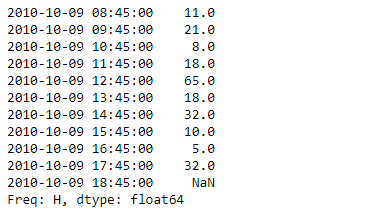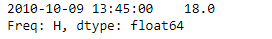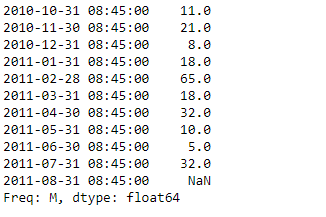# Python | Pandas Series.at_time()

• Last Updated : 17 Feb, 2019

Pandas series is a One-dimensional ndarray with axis labels. The labels need not be unique but must be a hashable type. The object supports both integer- and label-based indexing and provides a host of methods for performing operations involving the index.

Pandas` Series.at_time()` function is used to select values at particular time of day (e.g. 9:30AM) in the given series object.

Syntax: Series.at_time(time, asof=False, axis=None)

Parameter :
time : datetime.time or string
axis : {0 or ‘index’, 1 or ‘columns’}, default 0

Returns : values_at_time : same type as caller

Example #1: Use `Series.at_time()` function to return the values at particular time of the day in the given series object.

 `# importing pandas as pd ` `import` `pandas as pd ` ` `  `# Creating the Series ` `sr ``=` `pd.Series([``11``, ``21``, ``8``, ``18``, ``65``, ``18``, ``32``, ``10``, ``5``, ``32``, ``None``]) ` ` `  `# Create the Index ` `index_ ``=` `pd.date_range(``'2010-10-09 08:45'``, periods ``=` `11``, freq ``=``'H'``) ` ` `  `# set the index ` `sr.index ``=` `index_ ` ` `  `# Print the series ` `print``(sr) `

Output :Now we will use `Series.at_time()` function to return the values at particular time of the day in the given series object.

 `# return values at particular time of the day ` `result ``=` `sr.at_time(time ``=` `'13:45:00'``) ` ` `  `# Print the result ` `print``(result) `

Output :As we can see in the output, the `Series.at_time()` function has successfully returned the value at the particular time of the day in the given series object.

Example #2 : Use `Series.at_time()` function to return the values at particular time of the day in the given series object.

 `# importing pandas as pd ` `import` `pandas as pd ` ` `  `# Creating the Series ` `sr ``=` `pd.Series([``11``, ``21``, ``8``, ``18``, ``65``, ``18``, ``32``, ``10``, ``5``, ``32``, ``None``]) ` ` `  `# Create the Index ` `# apply monthly frequency ` `index_ ``=` `pd.date_range(``'2010-10-09 08:45'``, periods ``=` `11``, freq ``=``'M'``) ` ` `  `# set the index ` `sr.index ``=` `index_ ` ` `  `# Print the series ` `print``(sr) `

Output :Now we will use `Series.at_time()` function to return the values at particular time of the day in the given series object.

 `# return values at particular time of the day ` `result ``=` `sr.at_time(time ``=` `'08:45:00'``) ` ` `  `# Print the result ` `print``(result) `

Output :As we can see in the output, the `Series.at_time()` function has successfully returned the value at the particular time of the day in the given series object. All of the values in the series object has been returned as they are having the time value equal to the passed time.

My Personal Notes arrow_drop_up
Recommended Articles
Page :